# blog posts# Before performing any statistical test, you should make sure that your data is normally distributed. The SPSS Data Normality Test allows you to learn more about your data to choose the best test.

## How to test the normality of data distribution in SPSS

We need a series of statistical data to test the normality of data distribution. The table below deals with the two variables of age and gender, and half of the statistical population consists of men and the other half of women.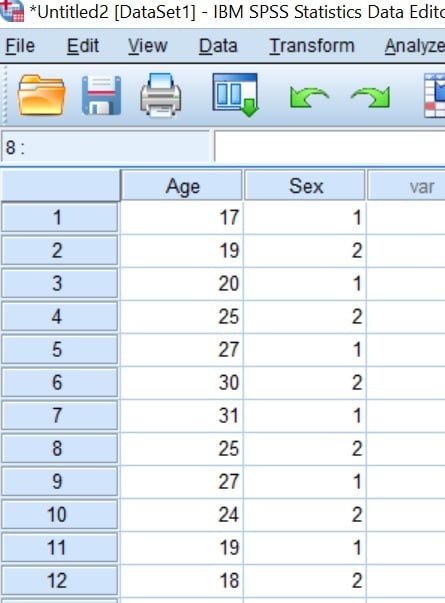## Execution of test for normality of data distribution

There are several different tests to determine the normal distribution of data in SPSS. If you have not done this test before, we suggest that you use the Shapiro-Wilk test to determine if the data distribution is normal:

1. First, go to Analyze> Descriptive Statistics> Explore .2. This will open the Explore window. To determine if the data distribution is normal, you must first enter the variable you want (eg age) in the Dependent List box.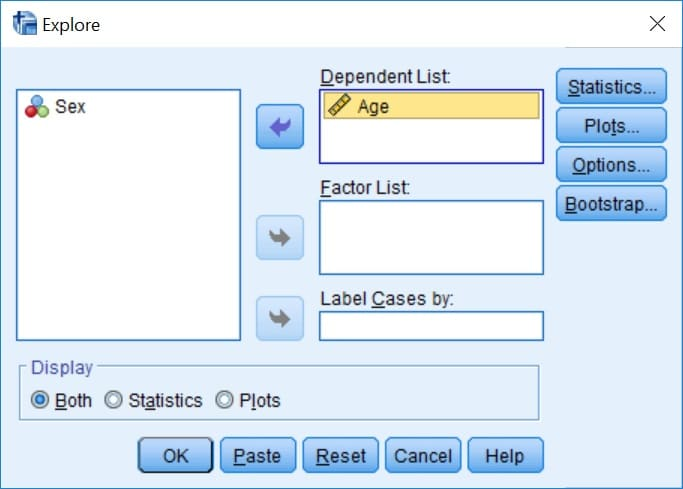3. Then click on Plot to open another window. In the window that opens, check Normality plot with tests and Histogram.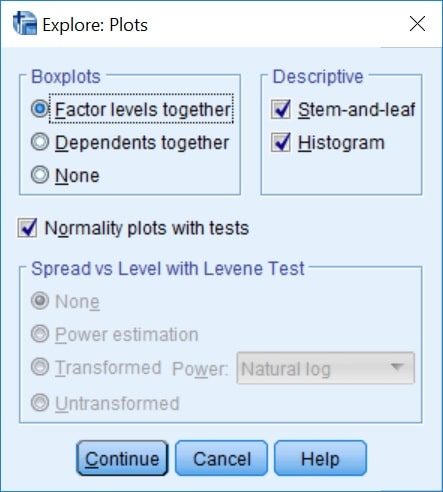4. Then click Continue in the Plot window and Ok in the Explore window.

## Test output

Now if you look at the output, you can get a lot of information. The first table (Case Processing Summary) provides information about the number of research samples and the next table (Descriptive) also provides information about data, mean, distance confidence and standard deviation. To see the result of the test of normality of data distribution, we need the Tests of Normality table.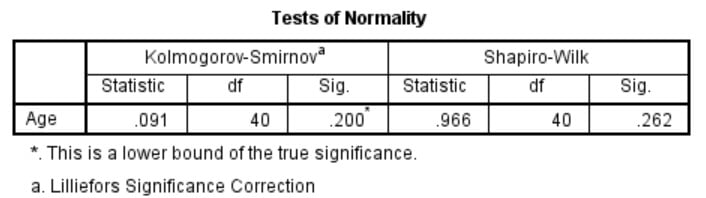SPSS software performs two different tests called Klomogorf-Smirnov and Shapiro-Wilk to test the normality of data distribution. In general, if the statistical population is small (2,000 people), we should use the Klomogorf-Smirnov test, and if it is large, we should use the Shapiro-Wilk test.

For this reason, in this example we use the results of the Shapiro-Wilk test.

• Statistics : value w
• df : degree of freedom in analysis
• Sig .: Significance level or probability value

If the value of sig. If it is less than 0.05, it means that the data is not normally distributed, and if it is greater than 0.05, it means that the data is normally distributed.

## Interpretation of results

In the example above, the value of sig. In the Shapiro-Wilk test, it is 0.262. Therefore, age-variable data in this sample are normally distributed.

## Results of normal data distribution in a group

Through the data normality test in SPSS, you can also decide on the normality of data distribution in a group.

To do this, repeat steps 1 and 2 according to the steps above. Then in the Factor List box, enter the name of the group you want (for example, gender).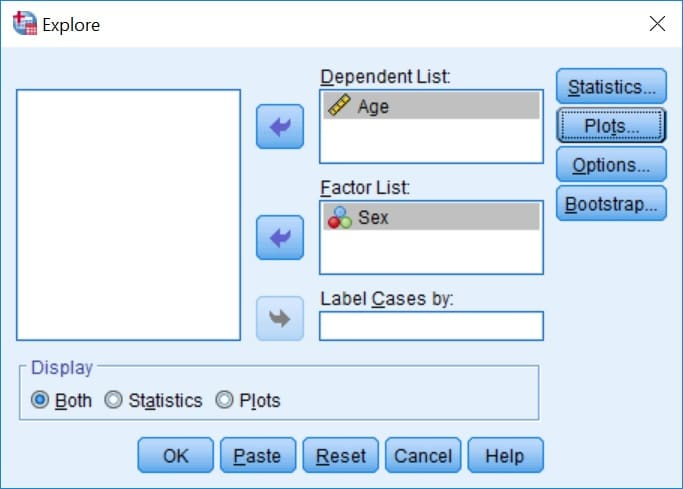This will separate the test results and output according to the group (male and female).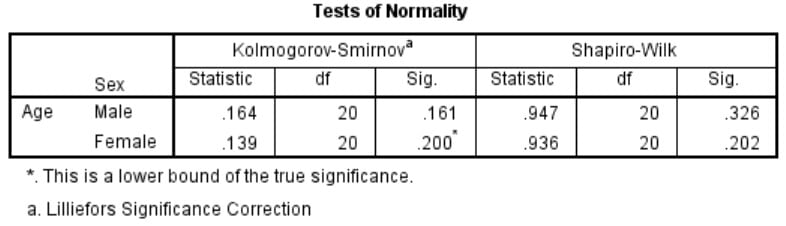Here too if the value of sig. If it is less than 0.05, it means that the data is not normally distributed, and if it is greater than 0.05, it means that the data is normally distributed.